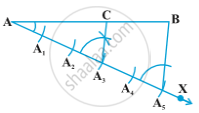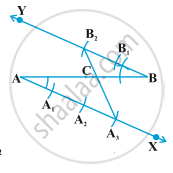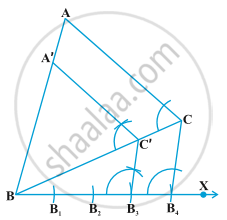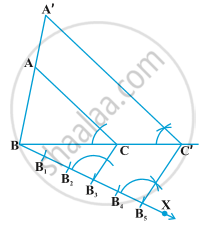Share

# Division of a Line Segment

#### description

• Division of Line Segment in a Given Ratio
• Construction of a Triangle Similar to a Given Triangle
• To divide a line segment in a given ratio
• To construct a triangle similar to a given triangle as per given scale factor

#### notes

Construction 1 : To divide a line segment in a given ratio.

Given a line segment AB, we want to divide it in the ratio m : n, where both m and n are positive integers. To help you to understand it, we shall take m = 3 and n = 2.

Steps of Construction :1)Draw a line AB.

2)Draw AX making an acute angle with AB.

3)Locate 5 (m + n) points "A"_1, "A"_2, "A"_3,"A"_4 and "A"_5 on AX with the help of compass, so that "A A"_1= "A"_1"A"_2 = "A"_2"A"_3 = "A"_3"A"_4 = "A"_4"A"_5.

4) Join "BA"_5.

5)Through the point "A"_3 (m = 3), draw a line parallel to "A"_5"B" intersecting AB at the point C. Since "A"_3"C" is parallel to "A"_5"B", therefore,

"AA"_3/("A"_3"A"_5)= "AC"/"CB"    (by basic proportionality theorem)

"AA"_3/("A"_3"A"_5)= 3/2    (by construction)

therefore, "AC"/"CB"= 3/2

This shows that C divides AB in the ratio 3 : 2.

Alternative Method

Steps of Construction :1. Draw any ray AX making an acute angle with AB.

2. Draw a ray BY || AX by making ABY equal to BAX.

3. Locate the points "A"_1, "A"_2, "A"_3 (m = 3) on AX and "B"_1, "B"_2  (n = 2) on BY such that "AA"_1 = "A"_1"A"_2 = "A"_2"A"_3  = "BB"_1 = "B"_1"B"_2

4. Join "A"_3"B"_2. Let it intersect AB at a point C

Then AC : CB = 3 : 2.

Construction 2- To construct a triangle similar to a given triangle as per given scale factor.

Example 1 : Construct a triangle similar to a given triangle ABC with its sides equal

to 3/4 of the corresponding sides of the triangle ABC.

Solution: Given a triangle ABC, we are required to construct another triangle whose

sides are 3/4 of the corresponding sides of the triangle ABC.

Steps of Construction :1) Draw ΔABC.

2) Draw ray BX making an acute angle with AB

3) Locate 4 equal points "B"_1 to "B"_4 on BX

4) Join "B"_4"C"

5) Draw a line through "B"_3 || "B"_4"C" to intersect BC at C′

6) Draw line through C′ || AC to intersect AB at A′

Then, ∆ A′BC′ is the required triangle.

Let us now see how this construction gives the required triangle.

"BC′"/"C′C"  = 3/1   (By construction)

Therefore, "BC"/"BC′" = ("BC′" + "C′ C")/"B C′" = 1+ "C′ C"/"B C′"

= 1+ 1/3= 4/3

i.e "B C′"/"BC"= 3/4

Also C′A′ is parallel to CA. Therefore, ∆ A′BC′ ~ ∆ ABC. (Angle-Angle rule)

So, "A′B"/"AB"= "A′C′"/"AC"= "BC′"/"BC"= 3/4

Example 2 : Construct a triangle similar to a given triangle ABC with its sides equal

to 5/3 of the corresponding sides of the triangle ABC .

Solution : Given a triangle ABC, we are required to construct a triangle whose sides

are 5/3 of the corresponding sides of ∆ ABC.

Steps of Construction :1) Draw ΔABC.

2) Draw ray BX making an acute angle with BC.

3) Locate 5 equal points "B"_1 to "B"_5 on BX

4) Join "B"_3"C"

5) Draw line through "B"_5 parallel to "B"_3"C" to intersect BC at C

6) Draw line through C′ parallel AC to intersect AB at A′

Then A′BC′ is the required triangle.

For justification of the construction, note that ∆ ABC ~ ∆ A′BC′ (Angle-Angle rule)

Therefore, "AB"/"A′B"= "AC"/"A′C′"= "BC"/"BC′"

But, "BC"/"BC′"= "BB"_3/"BB"_5= 3/5,

so, "BC′"/"BC"= 5/3, and, therefore,

"A′B"/"AB"= "A′C′"/"AC"= "BC′"/"BC"= 5/3

### Shaalaa.com

Constructions part 1 (Divide a line in a ratio) [00:09:39]
S
1
0%